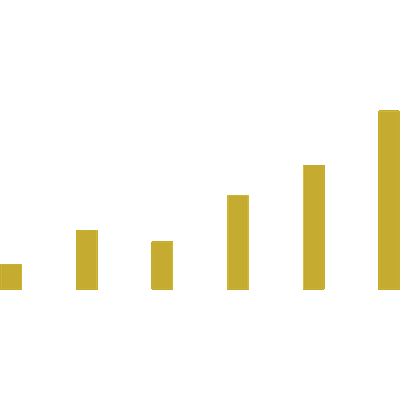# Registered Voter Economic Confidence IndexThe Registered Voter Economic Confidence Index (RVECI) is conducted monthly by Big Data Poll for the Public Polling Project. It includes interviews from at least 2,000 registered voters nationwide via online panel. The sampling error is ± 2.1% at a 95% confidence interval.

## Registered Voter Economic Confidence Index Methodology

Voters are asked to assign either a “positive”, “neutral”, or “negative” rating to reflect their perception for each of the five sub-indicators, which can be found in the interactive graphics layout above. Each sub-indicator level is calculated as a relative value by dividing the positive percentage by the sum of the positive and negative percentages.

For instance, here is “Current Business Conditions” for the index measured in August, 2021.

Twenty-five percent (25%) said they had a “positive” view of current business conditions and 30.8% said they had a “negative” view. So, let’s do the math.

25 / 55.8 (25+30.8) = .448

That just means 44.8%. Get it?

Let’s also do the math for “Current Employment Conditions”. This month, 28.7% said they had a positive view and 39.3% indicated they had a “negative” view. Again, let’s do the math.

28.7 / 68 (28.7+39.3) = .422

That is 42.2%.

After we have all five sub-indicator levels, we can calculate the two subindex levels and ultimately the main index level.

### Sub-Indicator Levels

Current Employment Conditions: 42.2
Expectations Employment Conditions: 54.2
Expectations Total Family Income: 50.2

The Present Situation Index is the average of the first two sub-indicator levels because they deal with views of the present situation, obviously. We did the prerequisite math above. The Expectations Index is the average of the last three sub-indicator levels because and measure the six-month outlook.

Remember “Order of Operations” in school? How about PEMDAS, or “Please Excuse My Dear Aunt Sally”?

1 – Parentheses
2 – Exponents and Roots
3 – Multiplication
4 – Division
6 – Subtraction

We first deal with the parentheses before dividing to calculate each subindex level.

### Present Situation Index (RED DASH)

(44.8 + 42.2) ÷ 2 = 43.5

### Expectations Index (BLUE DASH)

(51.4 + 54.2 + 50.2) ÷ 3 = 51.9

### Registered Voter Economic Confidence Index (GOLD SOLID)

(44.8 + 42.2 + 51.4 + 54.2 + 50.2) ÷ 5 = 48.6